Courses

# Test: Properties of Fluids - 1

## 10 Questions MCQ Test Fluid Mechanics | Test: Properties of Fluids - 1

Description
This mock test of Test: Properties of Fluids - 1 for Mechanical Engineering helps you for every Mechanical Engineering entrance exam. This contains 10 Multiple Choice Questions for Mechanical Engineering Test: Properties of Fluids - 1 (mcq) to study with solutions a complete question bank. The solved questions answers in this Test: Properties of Fluids - 1 quiz give you a good mix of easy questions and tough questions. Mechanical Engineering students definitely take this Test: Properties of Fluids - 1 exercise for a better result in the exam. You can find other Test: Properties of Fluids - 1 extra questions, long questions & short questions for Mechanical Engineering on EduRev as well by searching above.
QUESTION: 1

### What is the equivalent pressure head of mercury corresponding to 30 cm column of kerosene of relative density 0.8?

Solution: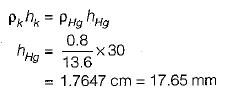QUESTION: 2

### What is dynamic viscosity in Pa-s for a liquid having relative density of 0.85 and kinematic viscosity of 2.5 centistoke?

Solution: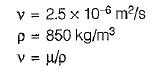or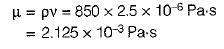QUESTION: 3

### The space between two parallel plates kept 4 mm apart is filled with an oil of dynamic viscosity 0.2 Pa-s. What is the shear stress on the lower fixed plate, if the upper one is moved with a velocity of 1.6 m/s? .

Solution: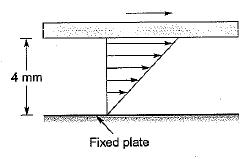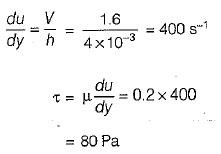QUESTION: 4

The velocity distribution in a viscous flow over a plate is given by
u = 4y - y2 for y ≤ 2m
where, u = velocity in m/s at a point distant y from the plate. If the coefficient of dynamic viscosity is 1.5 Pa-s, what is the shear stress at y= 1.2 m?

Solution: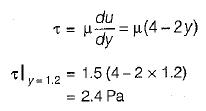QUESTION: 5

What is the pressure inside a soap bubble, over the atmospheric pressure if its diameter is 2 cm and the surface tension is 0.1 N/m?

Solution: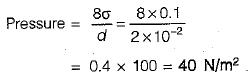QUESTION: 6

The gap between a -horizontal shaft and a concentric sleeve is filled with viscous oil. The sleeve moves with a constant velocity of 1.5 m/s when a force of 1500 N is applied parallel to the axis of the shaft. If it was required to move the sleeve at a velocity of 2 m/s, what should have been the force?

Solution: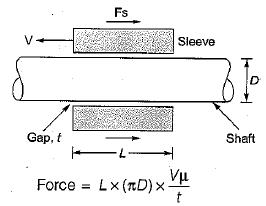for the given problem, L, D, μ and fare constant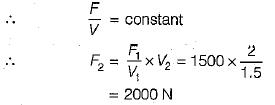QUESTION: 7

A perfect fluid is:

Solution:

A perfect fluid (also known as an ideal fluid) is Incompressible and frictionless.

QUESTION: 8

The concept of continuum in fluid flow assumes that the characteristics length of the flow is

Solution:
• The concept of the continuum is a kind of idealization of the continuous description of matter where the properties of the matter are considered as continuous functions of space variables.
• In this approach, the matter is continuously distributed with no void present continuum concept be applied if the mean free path is much less than the system dimensions.
• Although any matter is composed of several molecules, the concept of continuum assumes a continuous distribution of mass within the matter or system with no empty space, instead of the actual conglomeration of separate molecules.
• Describing a fluid flow quantitatively makes it necessary to assume that flow variables (pressure, velocity etc.) and fluid properties vary continuously from one point to another.
• One of the factors considered important in determining the validity of the continuum model is molecular density. It is the distance between the molecules which is characterized by a mean free path ( λ ). It is calculated by finding statistical average distance the molecules travel between two successive collisions.
• If the mean free path is very small as compared with some characteristic length in the flow domain (i.e., the molecular density is very high) then the gas can be treated as a continuous medium.
• If the mean free path is large in comparison to some characteristic length, the gas cannot be considered continuous and it should be analysed by the molecular theory.
• A dimensionless parameter is known as Knudsen number, Kn = λ/L, where λ is the mean free path and L is the characteristic length. It describes the degree of departure from the continuum.
• Usually when Kn > 0.01, the concept of the continuum does not hold good and when Kn < 0.01, the concept of the continuum does hold good.
• In the continuum approach, fluid properties such as density, viscosity, thermal conductivity, temperature, etc. can be expressed as continuous functions of space and time.
QUESTION: 9

When a shear stress is applied to a substance, it is found to resist it by static deformation, the  substance is:

Solution:
• solid can resist shear stress by a static deformation; a fluid cannot
• Any shear stress applied to a fluid, no matter how small, will result in motion of that fluid
• The fluid moves and deforms continuously as long as the shear stress is applied
• So, a fluid at rest must be in a state of zero shear stress
QUESTION: 10

The condition of ‘no slip’ at boundaries is applicable to:

Solution: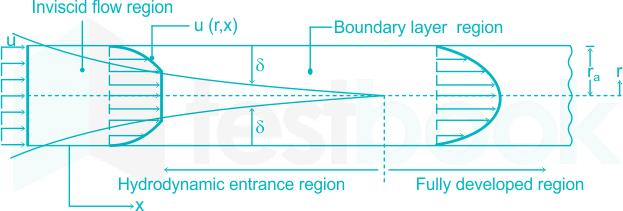When a real fluid flows past a solid boundary, a layer of fluid which comes in contact with the boundary surface adheres to it on account of viscosity. Since this layer of fluid cannot slip away from the boundary surface it attains the same velocity as that of the boundary.

In other words, at the boundary surface there is no relative motion between the fluid and the boundary. This condition is known as the no slip condition.

∴ The condition of "No-slip" at rigid boundaries is applicable to flow if all real fluids.